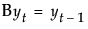t is the time index
B is the backshift operator defined asμ is the intercept or mean term
The constant estimate δ is given by the relation:
In the case of Seasonal ARIMA modeling, the differencing, autoregressive, and moving average operators are the product of seasonal and nonseasonal polynomials:
where s is the number of observations per period. The first index on the coefficients is the factor number (1 indicates nonseasonal, 2 indicates seasonal) and the second is the lag of the term.

Help created on 9/19/2017